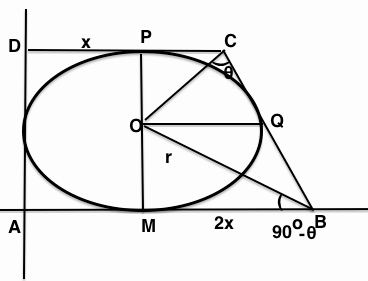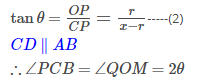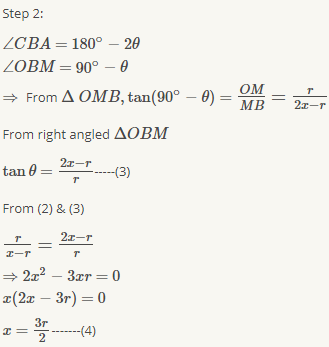Courses

# MCQ: Trigonometry, MAthematics JEE JEE Notes | EduRev

Created by: Dhruv

## JEE : MCQ: Trigonometry, MAthematics JEE JEE Notes | EduRev

The document MCQ: Trigonometry, MAthematics JEE JEE Notes | EduRev is a part of JEE category.
All you need of JEE at this link: JEE

LET ABCD be a quadrilateral with area 18 ,with side AB parallel to the side CD and AB=2CD .Let AD be perpendicular to AB and CD .If a circle is drawn inside the quadrilateral ABCD touching all the side ,then it's radius is A 3 B 2 C 3/2 D 1?Let CD=x then AB=2CD=2x.Let r be the radius of the circle inscribed in the quadrilateral ABCD.

Given: Area of quadrilateral ABCD=18 and ABis|| to CD.

⇒ 1/2(x+2x).2r=18

⇒ 3xr=18

⇒ xr=6-----(1)

OP=OM=PD=OQ=AM=r

⇒ PC=x−randMB=2x−r

Let ∠PCO=angleOCQ=θ then from right-angled ΔOPCFrom (1) & (4) we get,

xr=6

3rr^2=6

r^2=4

r=2

Hence (b) is the correct answer.

Offer running on EduRev: Apply code STAYHOME200 to get INR 200 off on our premium plan EduRev Infinity!

,

,

,

,

,

,

,

,

,

,

,

,

,

,

,

,

,

,

,

,

,

,

,

,

;